Vignette 5
Graph Theory and the Bridges of Königsberg

Königsberg was a city in Prussia situated on the Pregel River, which served as the residence of the dukes of Prussia in the 16th century.  (Today, the city is named Kaliningrad, and is a major industrial and commercial center of western Russia.)  The river Pregel flowed through the town, creating an island, as in the following picture.  Seven bridges spanned the various branches of the river, as shown.The Bridges of Königsberg

A famous problem concerning Königsberg was whether it was possible to take a walk through the town in such a way as to cross over every bridge once, and only once.  An example of a failed attempt to take such a walking tour is shown below.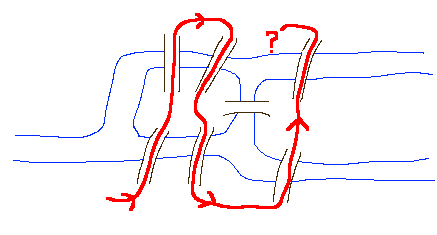OK, so this attempt didn't work.  But might there be some other path that would cross every bridge exactly once?  This problem was first solved by the prolific Swiss mathematician Leonhard Euler (pronounced "Oiler"), who invented the branch of mathematics now known as graph theory in the process of his solution.

Graphs

Euler's approach was to regard the spots of land (there are 4 of them) as points to be visited, and the bridges as paths between those points.  The mathematical essentials of the map of Königsberg can then be reduced to the following diagram, which is an example of what is called a graph: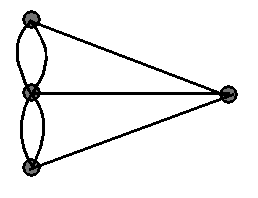A graph is a figure consisting of points (called vertices--the plural of vertex) and connecting lines or curves (called edges).  The problem of the bridges of Königsberg can then be reformulated as whether this graph can be traced without tracing any edge more than once.

For each of the vertices of a graph, the order of the vertex is the number of edges at that vertex.  The figure below shows the graph of the Königsberg bridge problem, with the orders of the vertices labeled.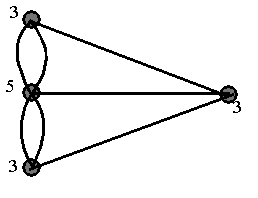Euler's Solution

Euler's solution to the problem of the Königsberg bridges involved the observation that when a vertex is "visited" in the middle of the process of tracing a graph, there must be an edge coming into the vertex, and another edge leaving it; and so the order of the vertex must be an even number.  This must be true for all but at most two of the vertices--the one you start at, and the one you end at, and so a connected graph is traversible if and only if it has at most two vertices of odd order.  (Note that the starting and ending vertices may be the same, in which case the order of every vertex must be even.)  Now a quick look at the graph above shows that there are more than two vertices of odd order, and so the graph cannot be traced; that is the desired walking tour of Königsberg is impossible.

Additional Fun with Graphs

1.  Suppose the citizens of Königsberg decided to build an eighth bridge, as in the diagram shown below.  Would a walking tour of Königsberg now be possible?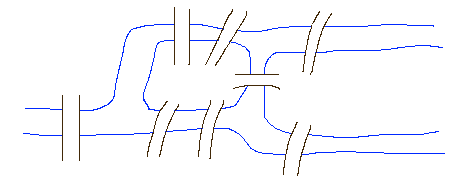2.  Show how you could add a ninth bridge to the diagram above, to make the walking tour once again impossible.

A Different Problem with the Same Solution

Euler's solution can also be applied to problems that at first look different from the problem of the Königsberg bridges.  Consider the problem of whether it is possible to draw a continuous curve that passes through each of the ten edges (line segments) of the following figure exactly once.  (A curve that passes through a vertex is not allowed.)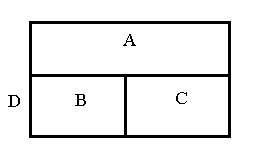The next figure shows a start on a possible solution.Is there a systematic way to approach this problem?  To analyze this problem, we will create a graph with four vertices, one for each of the four regions (including the outside region, D).  There will be ten edges in our graph, one for each of the boundary edges between two of the regions.  For instance, our graph will have three edges between the vertices for regions A and D, because there are three boundary edges between regions A and D in the figure above.  The resulting graph can be drawn as follows: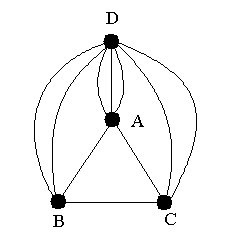The question of whether there is a continuous curve passing through all ten edges is equivalent to the question of whether this graph can be traced.  Since there are only two vertices of odd order, Euler's theorem not only answers the question in the affirmative, but also tells you that you must start either in region A or in region D.

Can you determine whether it is possible to draw a continuous curve that passes through each of the edges of the following figure exactly once?  Now that you know the secret, you can easily make up your own similar challenges.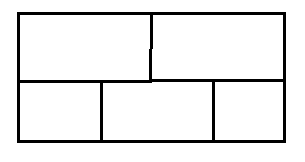Graph Theory Today

Today, graph theory is a highly developed field of mathematics, and is both a fertile ground for the creation of new mathematics and an area with many, many applications.  Many research problems in graph theory are easily stated and easily understood (although perhaps not easily solved).  A few of the applications of graph theory include transportation and warehousing applications, planning and scheduling, analysis of electrical networks, and even understanding the Internet!

Further Exploration

• Games and Their Graphs
• The Beginnings of Topology
• Richard J. Trudeau, Dots and Lines.  In JCU Library, call number QA166.T74
• Sherman K. Stein, Mathematics: The Man-Made Universe.  In JCU Library, call number QA93 .S68
• Solomon Garfunkel, ed., For All Practical Purposes.  In JCU Library, call number QA7 .F68

Copyright © 2000 by Carl R. Spitznagel